##### Quantum Physics For Dummies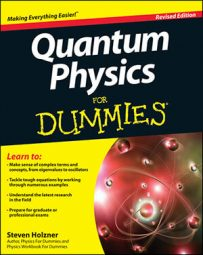Quantum physics experiments take place in the lab frame, but you do scattering calculations in the center-of-mass frame, so you have to know how to relate the angle between the two frames.

Here's how this works: The following figure shows scattering in the lab frame.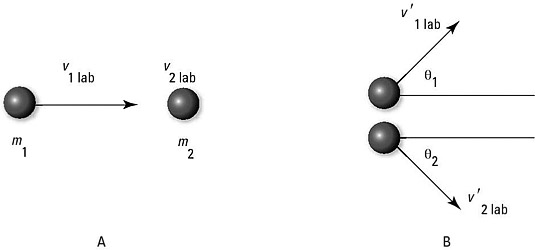Scattering in the lab frame.

One particle, traveling at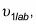is incident on another particle that's at rest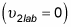and hits it. After the collision, the first particle is scattered at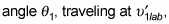and the other particle is scattered at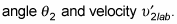Now in the center-of-mass frame, the center of mass is stationary and the particles head toward each other. After they collide, they head away from each other at angles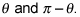You have to move back and forth between these two frames — the lab frame and the center-of-mass frame — so you need to relate the velocities and angles (in a nonrelativistic way).

To relate the angles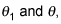you start by noting that you can connect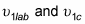using the velocity of the center of mass,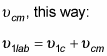In addition, here's what you can say about the velocity of particle 1 after it collides with particle 2: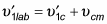Now you can find the components of these velocities: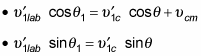Dividing the equation in the second bullet by the one in the first gives you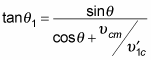But wouldn't it be easier if you could relate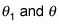by something that didn't involve the velocities, only the masses, such as the following?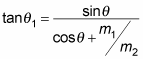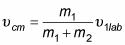And you can show that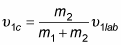You can also use the conservation of momentum to say what happens after the collision. In fact, because the center of mass is stationary in the center-of-mass frame, the total momentum before and after the collision is zero in that frame, like this: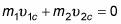Therefore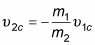And after the collision,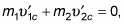which means that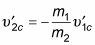Also, if the collision is elastic, kinetic energy is conserved in addition to momentum, so that means the following is true: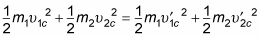Substituting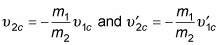into this equation gives you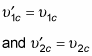Given these two equations, you can redo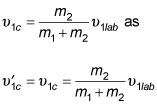Dividing the magnitude of each side of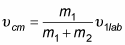by the magnitude of the above equation gives you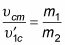And because you saw earlier thatsubstitutinginto this equation gives you at lastOkay, that relateswhich is what you were trying to do. Using the relation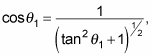you can rewriteas the following: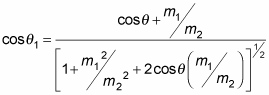You can also relate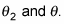You can show that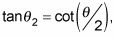which, using a little trig, means that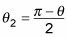You've now related the angles between the lab and center-of-mass frames.